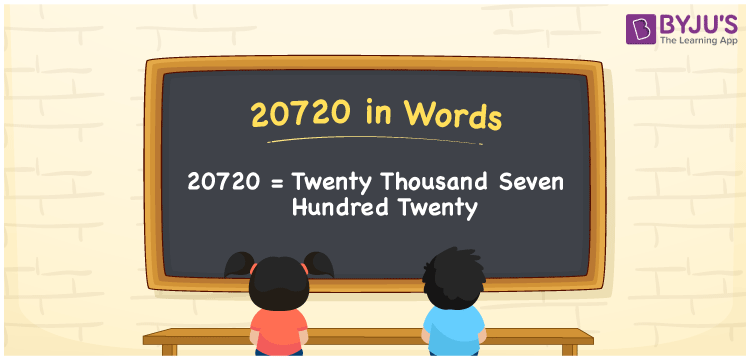# 20720 in words

20720 in words is written as Twenty Thousand Seven Hundred and Twenty. In 20720, 2 has a place value of ten thousand, 7 is in the place value of hundred and next 2 is in the place value of ten. The article on Place Value gives more information. The number 20720 is used in expressions that relate to money, distance, social media views, and many more. For example, “The postal code for Maryland USA, is Twenty Thousand Seven Hundred and Twenty.”

 20720 in words Twenty Thousand Seven Hundred and Twenty Twenty Thousand Seven Hundred and Twenty in Numbers 20720

## 20720 in English Words## How to Write 20720 in Words?

We can convert 20720 to words using a place value chart. The number 20720 has 5 digits, so let’s make a chart that shows the place value up to 5 digits.

 Ten thousand Thousands Hundreds Tens Ones 2 0 7 2 0

Thus, we can write the expanded form as:

2 × Ten thousand + 0 × Thousand + 7 × Hundred + 2 × Ten + 0 × One

= 2 × 10000 + 0 × 1000 + 7 × 100 + 2 × 10 + 0 × 1

= 20720.

= Twenty Thousand Seven Hundred and Twenty.

20720 is the natural number that is succeeded by 20719 and preceded by 20721.

20720 in words – Twenty Thousand Seven Hundred and Twenty.

Is 20720 an odd number? – No.

Is 20720 an even number? – Yes.

Is 20720 a perfect square number? – No.

Is 20720 a perfect cube number? – No.

Is 20720 a prime number? – No.

Is 20720 a composite number? – Yes.

## Solved Example

1. Write the number 20720 in expanded form

Solution: 2 x 10000 + 0 x 1000 + 7 x 100 + 2 x 10 + 0 x 1

Or Just 2 x 10000 + 7 x 100 + 2 x 10

We can write 20720 = 20000 + 0000 + 700 + 20 + 0

= 2 x 10000 + 0 x 1000 + 7 x 100 + 2 x 10 + 0 x 1.

## Frequently Asked Questions on 20720 in words

Q1

### How to write the number 20720 in words?

20720 in words is written as Twenty Thousand Seven Hundred and Twenty.
Q2

### State whether True or False. 20720 is divisible by 3?

False. 20720 is not divisible by 3.
Q3

### Is 20720 divisible by 10?

Yes. 20720 is divisible by 10.